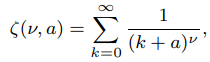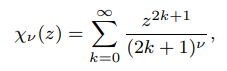# Special Functions: Simple Definition, Examples

Contents:

## What are Special Functions?

Special functions are well-known, classical functions. Many are named after the person who first studied it. For example, the Bessel Function is named after the astronomer Friedrich Bessel (1784-1846). Most of these functions have been used for centuries, although several dozen have been formulated in recent years.

Paul Turan (as cited in Andrews et. al, 1999) once said that a more suitable name for these types of functions is “useful functions.” You can also think of them as commonly used functions, or elementary functions—ones that are building blocks for other function types.

## What Functions are “Special”?

In order for a function to be defined as “special,” it also has to be useful in a practical sense, especially in finding solutions for differential equations. For example, Bessel functions, which deal with circular or spherical symmetry, are useful for studying heat flow. In order to grasp the use of these functions, you’ll usually have to study multivariable calculus and differential equations as prerequisites.

## Common Special Functions

Perhaps surprisingly, special functions do not include those you’ll come across in the first semester or two of calculus, like power functions, logarithm function, and exponential functions. Although they are certainly “classical” functions that have been around for centuries, they aren’t very useful for solving physical problems. For that, you need more complex functions. Some authors do include the trig functions (cosine function, sine function, etc.) in the definition of special functions as a group (as opposed to individual functions, which are deemed as too simplistic on their own).

There are many more. In fact, dozens of books have been written about special functions (you can find two in the references below). For definitions of specific function types, see: Types of Functions. You can even take an entire university course on them.

## What is the Chi Function?

The Chi Function (also called Legendre’s Chi Function) is a special function defined with the following formulas:0 < a ≤ 1, Re ν > 1,

And|z| ≤ 1, Re ν > 1 with ν = 2, 3, 4, …
Where “re” stands for Real.

The second formula is usually called the Legendre chi function of order n. The function’s Taylor series is also a Dirichlet series. It is a special case of the Lerch transcendent.

## Relation to Polylogarithm

The chi function resembles the Dirichlet series for the polylogarithm. It is related to the polylogarithm function for integral ν = 2, 3 by:## History of the Chi Function

Adrien-Marie Legendre studied the chi function in 1811 (in Exercices de calcul integral) (1811) and used the letter phi(φ) instead of chi(χ) to denote it. The use of χ dates to Edward’s 1889 text A Treatise on the Integral Calculus.

## Linear combination of Special Functions: The Meijer G-Function

The Meijer G-Function is a very general family of functions, usually defined by the following complex line integral:Where Γ = the gamma function.

Each G-function is a linear combination of special functions. These include as special cases:

In fact, most special functions and products of special functions are either G-functions or can be represented by products of G-functions with elementary functions.

The G-function can also be extended to reproduce a wide variety of smooth functions, including exponential functions, trigonometric functions and many others.

## Uses for the Meijer G-function

The Meijer G-function has greater flexibility for choices of parameters in comparison to other special functions, which makes it a popular choice for finding solutions to a wide variety of problems. In addition, some problematic integrals—which could previously only be represented numerically—can be evaluated in closed form with use of the G-function.

The Meijer G-Function has many practical applications to many areas, where the function can represent solutions for a wide variety of problems. Fields that use the Meijer G-function extensively include:

• Generalized birth and death processes,
• Mathematical physics (hydrodynamics, potential theory, theory of elasticity etc.),
• Mathematical statistics,
• Optimization theory,
• Queuing theory,
• Sinusoidal signals,
• Theoretical physics,

## Struve Function

The Struve function is an uncommon special function defined by:Where:

While the function definition might seem a little complicated, you’ll rarely have to solve it. Most popular mathematical software includes it as a built in function. For example, in Maple, the function is StruveH. There are a few online calculators, including this one that calculates the modified Struve function, Lv(x).

## Notation for the Struve Function

The Struve function is generally denoted by Hv(x) or Hn(x). Occasionally you may see the “H” bolded (Hv(x)) or in lowercase hv(x), sometimes to distinguish it from Hermite polynomials. The modified Struve is usually denoted with an L instead of H.

## Use as Solutions to Bessel Differential Equations

Developed by H. Struve in 1882, the function is a solution to the non-homogeneous (or inhomogeneous) Bessel differential equation. Two versions of the function exist; Each version solves a different form of the inhomogenous Bessel equation:The Struve function solves the inhomogenous Bessel equation (1). A modified Struve solves the Bessel equation on the bottom (2).

Struve functions are generalized by the hypergeometric and Meijer G functions.

## Uses

The Struve function can be used for various calculations in mathematical physics. For example, to describe thin disk vibration and for various purposes in the theory of electromagnetism.

## References

Abramowitz, M. & Stegun, C. (Eds.). Sine and Cosine Integrals. Handbook of Mathematical Functions with Formulas, Graphs, and Mathematical Tables. 9th edition.
Andrews, G. et al. (1999). Special Functions. Cambridge University Press.
Baricz et. al (2018). Series of Bessel and Kummer-Type Functions. Springer.
Beals, R. (2013). Meijer G-Functions: A Gentle Introduction. Notices of the American Mathematical Society. Retrieved December 1, 2019 from: https://www.academia.edu/26546005/Meijer_G-Functions_A_Gentle_Introduction
Bell, W. (2013). Special Functions for Scientists and Engineers. Courier Corporation.
Cvijovic, D. and Klinowski, J. (1995). Closed-Form Summation of Some Trigonometric Series. Math. Comput. 64, 205-210.
Cvijovic, D. and Klinowski, J. (1999). Values of the Legendre Chi and Hurwitz Zeta Functions at Rational Arguments. Mathematics of Computation. Volume 68, Number 228, Pages 1623–1630. Retrieved November 21, 2019 from: https://www.ams.org/journals/mcom/1999-68-228/S0025-5718-99-01091-1/S0025-5718-99-01091-1.pdf
Cvijovic, D. (2006). Integral representations of the Legendre chi. J. Math. Anal. Appl. 332 (2007) 1056–1062
Kiryakova, V. (1993). Generalized Fractional Calculus and Applications. CRC Press.
Lewin, L. (1981). Polylogarithms and Associated Functions. Elsevier Science Ltd.
Luke, Y. (1969). Special Functions and Their Approximations:, Volume 2. Academic Press.
Maplesoft. StruveH, StruveL. Retrieved December 2, 2019 from: https://www.maplesoft.com/support/help/Maple/view.aspx?path=StruveH
Narcowish, F. (2005). Notes on…
Retrieved November 27, 2019 from: https://www.math.tamu.edu/~francis.narcowich/psfiles/special_fun.pdf
Oldham, K. et al. (2010). An Atlas of Functions: with Equator, the Atlas Function Calculator. Springer Science and Business Media.
Rojas, S. et al. (2015). Learning SciPy for Numerical and Scientific Computing – Second Edition. Packt Publishing Ltd.
Toth, V. (2007). Maple and Meijer’s G-function: a numerical instability and a cure. Retrieved December 1, 2019 from: vttoth.com/CMS/index.php/technical-notes/67

CITE THIS AS:
Stephanie Glen. "Special Functions: Simple Definition, Examples" From StatisticsHowTo.com: Elementary Statistics for the rest of us! https://www.statisticshowto.com/special-functions-simple-definition/